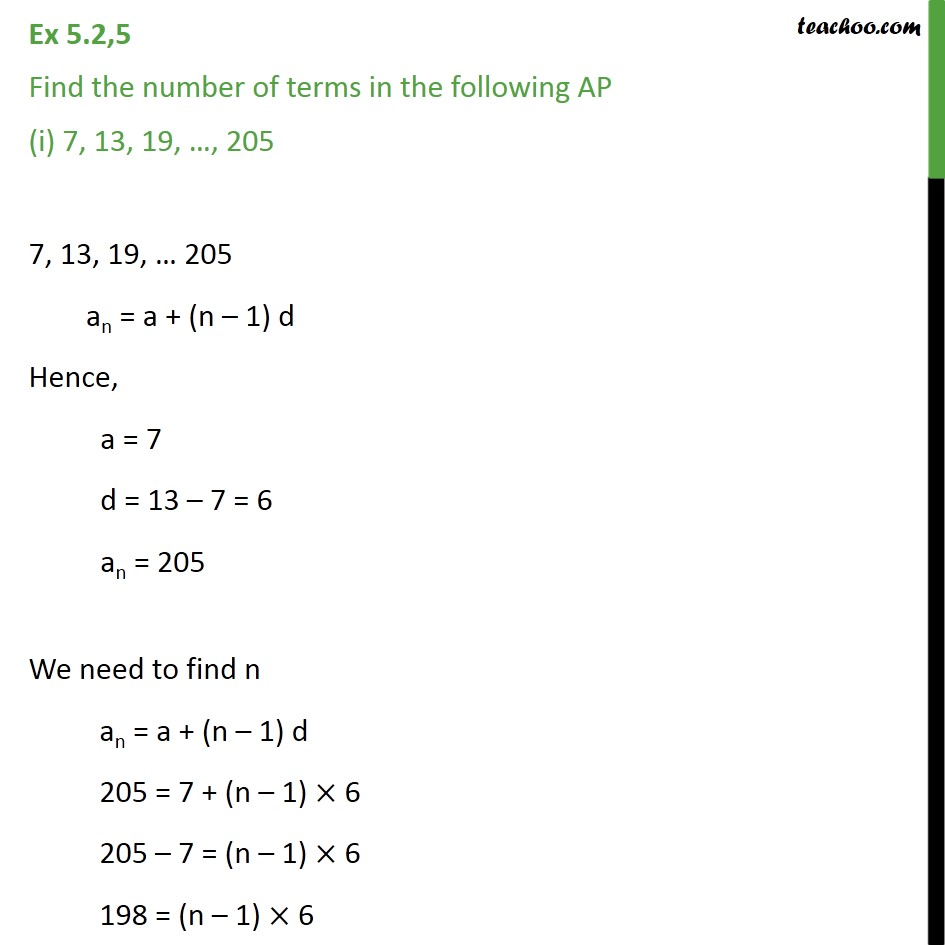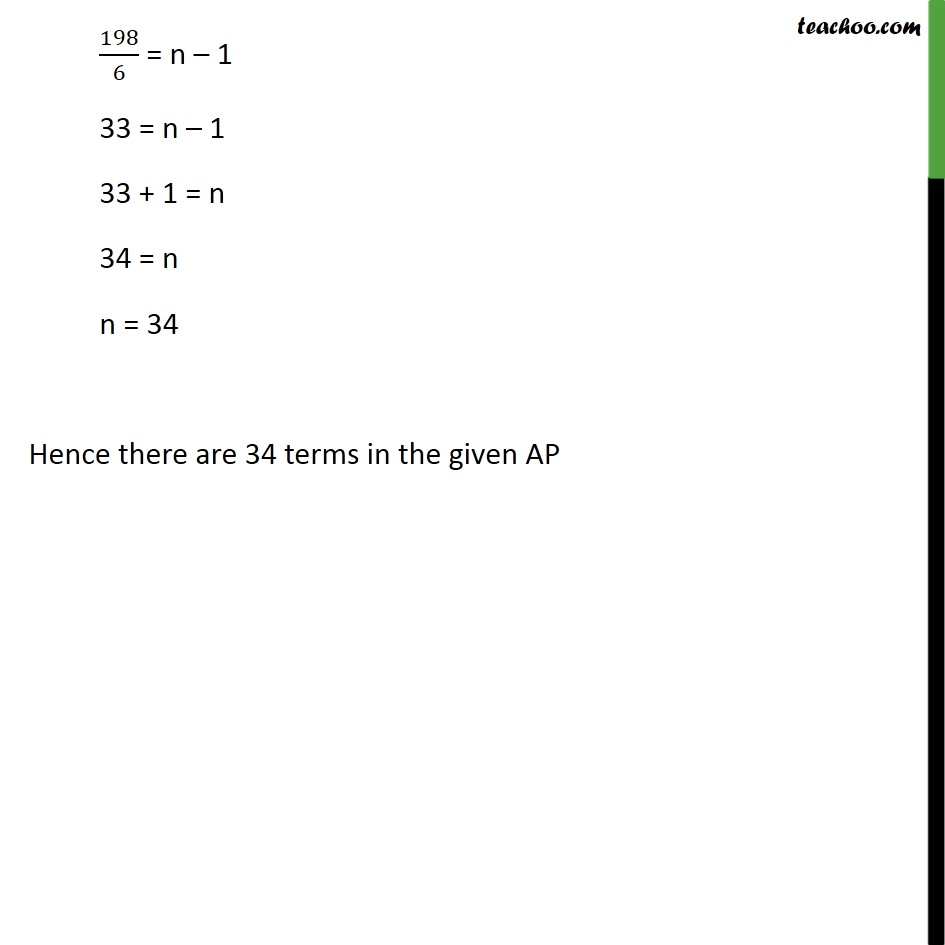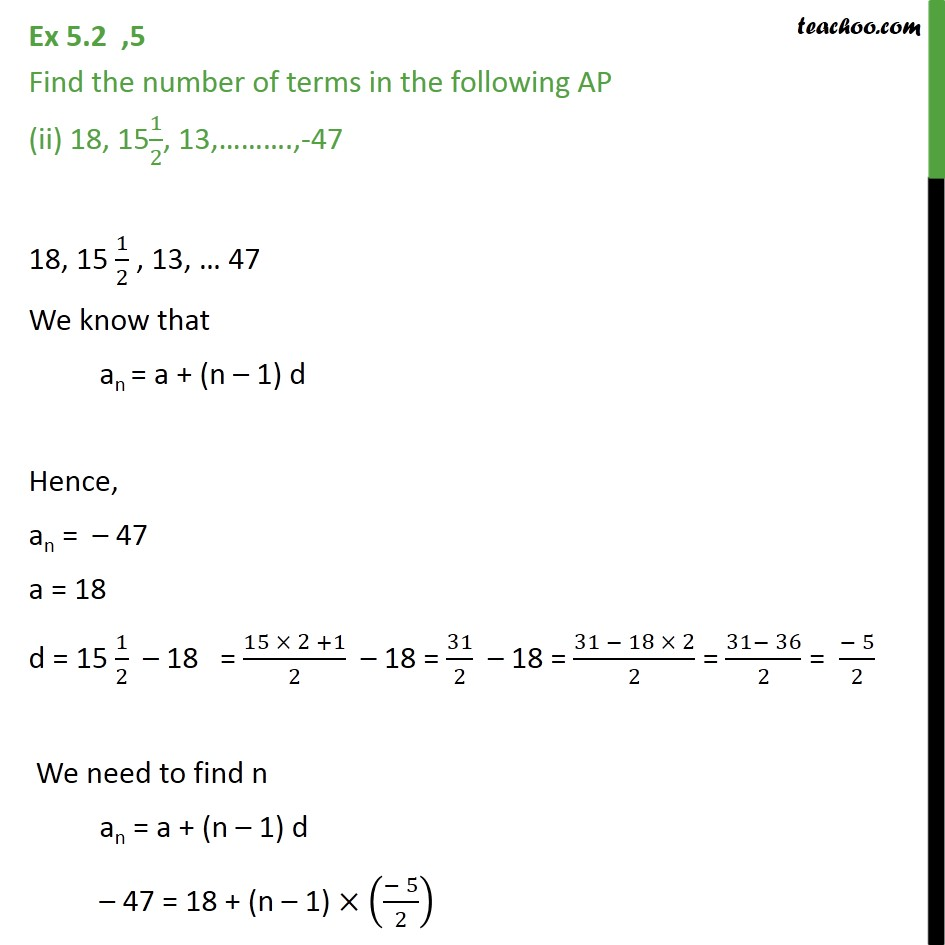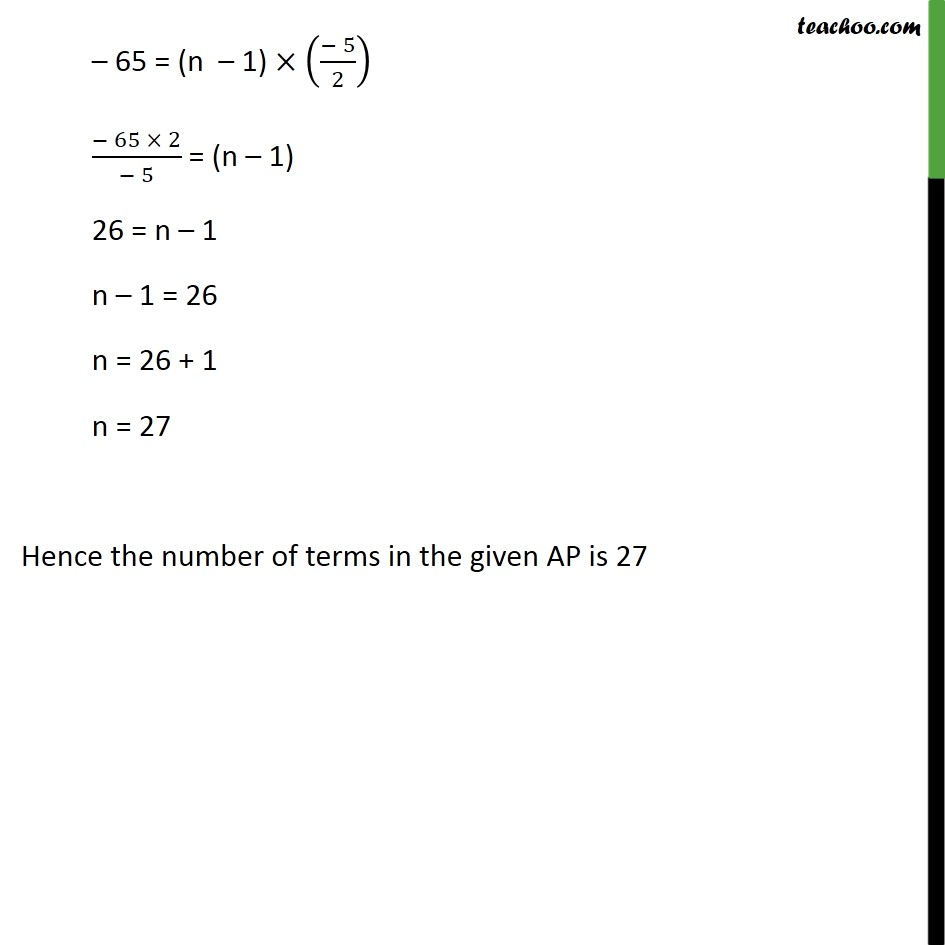Learn all Concepts of Chapter 5 Class 10 (with VIDEOS). Check - Arithmetic Progressions - Class 101. Chapter 5 Class 10 Arithmetic Progressions
2. Serial order wise
3. Ex 5.2

Transcript

Ex 5.2,5 Find the number of terms in the following AP (i) 7, 13, 19, , 205 7, 13, 19, 205 an = a + (n 1) d Hence, a = 7 d = 13 7 = 6 an = 205 We need to find n an = a + (n 1) d 205 = 7 + (n 1) 6 205 7 = (n 1) 6 198 = (n 1) 6 198/6 = n 1 33 = n 1 33 + 1 = n 34 = n n = 34 Hence there are 34 terms in the given AP Ex 5.2 ,5 Find the number of terms in the following AP (ii) 18, 151/2, 13, .,-47 18, 15 1/2 , 13, 47 We know that an = a + (n 1) d Hence, an = 47 a = 18 d = 15 1/2 18 = (15 2 +1)/2 18 = 31/2 18 = (31 18 2)/2 = (31 36)/2 = ( 5)/2 We need to find n an = a + (n 1) d 47 = 18 + (n 1) (( 5)/2) 65 = (n 1) (( 5)/2) ( 65 2)/( 5) = (n 1) 26 = n 1 n 1 = 26 n = 26 + 1 n = 27 Hence the number of terms in the given AP is 27

Ex 5.2